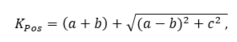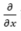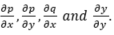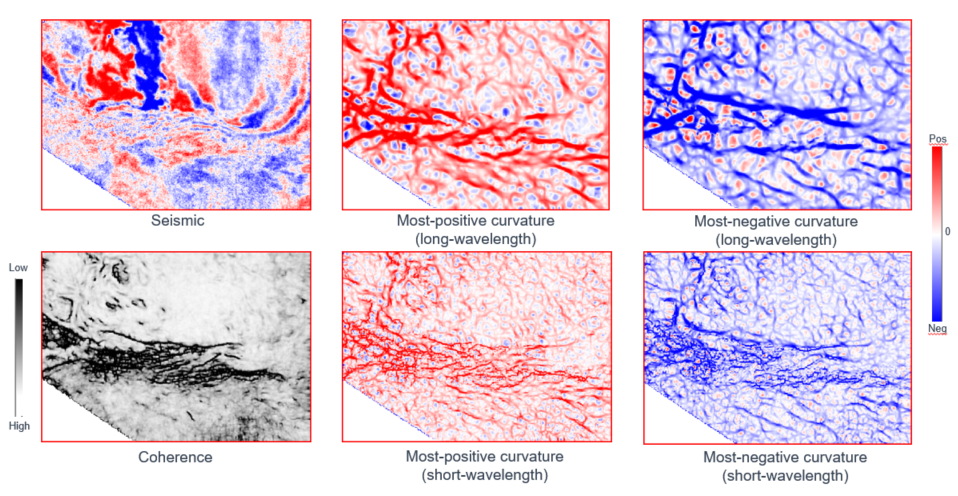# Curvature

## This suggests that in the simplest way one could calculate curvature by computing the first and second derivatives of the x- and y- components of the surface.

The surface computation of curvature involves fitting a quadratic surface to the mapped horizon using a least-squares regression and using nine sample points (eight neighbors around a given point). When nine sample points are used for the computation of curvature, it results in an overdetermined system when the solution is sought by the least squares regression method.  Different measures of curvature can then be written in terms of the six coefficients, as was shown by Roberts (2001).

For extension to volumetric computation of curvature, inline and crossline components of dip are computed, which Al-Dossary and Marfurt (2006) make use of to evaluate the derivatives numerically in the polynomial equation.

Of all the available curvature measures, Chopra and Marfurt (2007) recommend the application of the most-positive curvature and most-negative curvature attributes, for they are the easiest to understand intuitively.

Roberts (2001) has given the following expression for computation of the most-positive curvature.where a, b and c are the coefficients of the polynomial equation.

As we note above, curvature is a function of the first derivatives of the inline dip, p, and crossline dip, q.  In the method of Fourier filtering, Al-Dossary and Marfurt (2006) introduce a fractional index (α) based multispectral estimation of curvature. The derivative inin the Fourier domain is equivalent to multiplying the spectrum by (ik), but Al-Dossary and Marfurt (2006) introduce a fractional index α such that the spectrum gets multiplied by it. The smaller values of α yield the long-wavelength estimates of curvature, and larger values the short wavelength.  The inverse Fourier transform allows the generation of 3D convolution operator, which is convolved with p and q to obtain filtered versions ofBoth such estimates have their applications, long wavelength suitable for obtaining the gross definitions of the geometrical features and the short wavelength for extracting the finer details and thus more resolved images.Notice the clear set of faults/fractures generated from coherence and curvature attributes